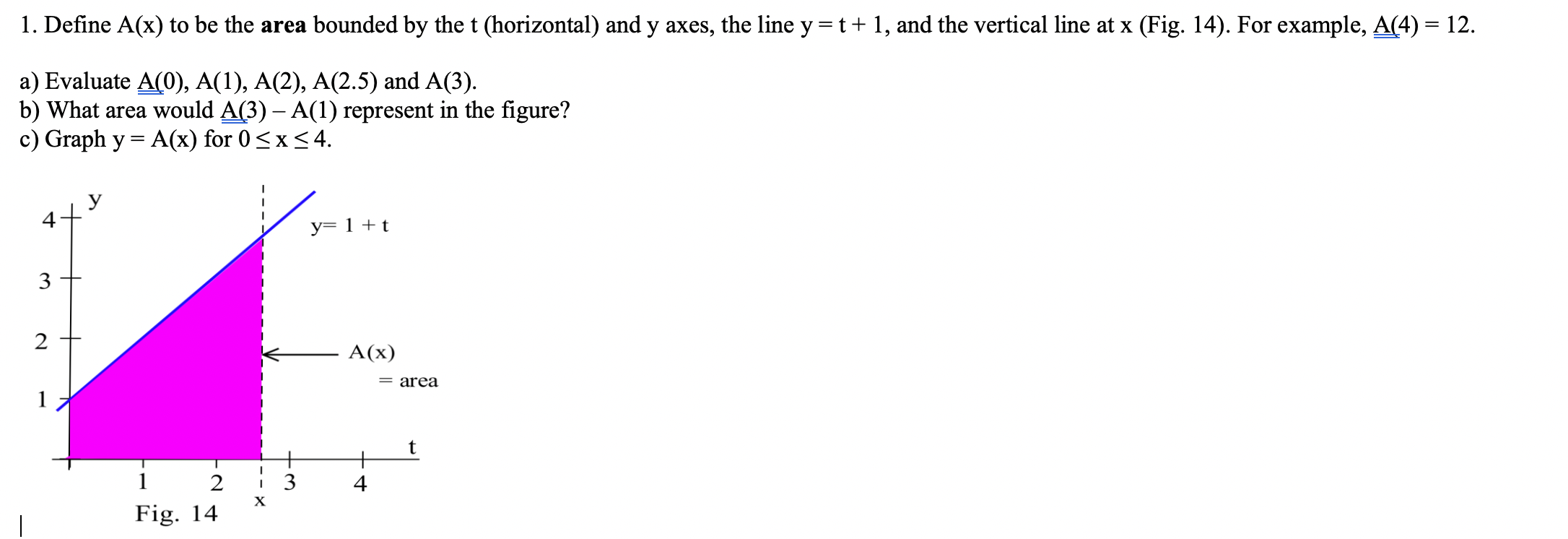# Question Solved1 Answer1. Define A(x) to be the area bounded by the t (horizontal) and y axes, the line y=t+1, and the vertical line at x (Fig. 14). For example, A(4) = 12. a) Evaluate A(0), A(1), A(2), A(2.5) and A(3). b) What area would A(3) - A(1) represent in the figure? c) Graph y= A(x) for 0 < x < 4. AXY y=1t < A(x) = area X Fig. 14Transcribed Image Text: 1. Define A(x) to be the area bounded by the t (horizontal) and y axes, the line y=t+1, and the vertical line at x (Fig. 14). For example, A(4) = 12. a) Evaluate A(0), A(1), A(2), A(2.5) and A(3). b) What area would A(3) - A(1) represent in the figure? c) Graph y= A(x) for 0 < x < 4. AXY y=1t < A(x) = area X Fig. 14
More
Transcribed Image Text: 1. Define A(x) to be the area bounded by the t (horizontal) and y axes, the line y=t+1, and the vertical line at x (Fig. 14). For example, A(4) = 12. a) Evaluate A(0), A(1), A(2), A(2.5) and A(3). b) What area would A(3) - A(1) represent in the figure? c) Graph y= A(x) for 0 < x < 4. AXY y=1t < A(x) = area X Fig. 14